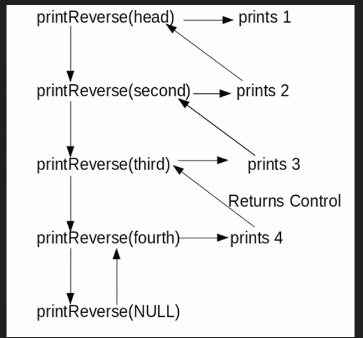GeeksforGeeks App
Open AppBrowser
Continue

# C# Program For Printing Reverse Of A Linked List Without Actually Reversing

Given a linked list, print reverse of it using a recursive function. For example, if the given linked list is 1->2->3->4, then output should be 4->3->2->1.
Note that the question is only about printing the reverse. To reverse the list itself see this
Difficulty Level: RookieAlgorithm:

```printReverse(head)
1. call print reverse for head->next

Implementation:

## C#

 `// C# program to print reverse``// of a linked list``using` `System;` `public` `class` `LinkedList``{``    ``// Head of list``    ``Node head;` `    ``// Linked list Node``    ``class` `Node``    ``{``        ``public` `int` `data;``        ``public` `Node next;``        ``public` `Node(``int` `d)``        ``{``            ``data = d; next = ``null``;``        ``}``    ``}` `    ``// Function to print reverse``    ``// of linked list``    ``void` `printReverse(Node head)``    ``{``        ``if` `(head == ``null``) ``return``;` `        ``// print list of head node``        ``printReverse(head.next);` `        ``// After everything else is printed``        ``Console.Write(head.data + ``" "``);``    ``}` `    ``// Utility Functions` `    ``// Inserts a new Node at front``    ``// of the list.``    ``public` `void` `push(``int` `new_data)``    ``{``        ``/* 1 & 2: Allocate the Node &``                  ``Put in the data*/``        ``Node new_node = ``new` `Node(new_data);` `        ``// 3. Make next of new Node as head``        ``new_node.next = head;` `        ``// 4. Move the head to point to``        ``// new Node``        ``head = new_node;``    ``}` `    ``// Driver code``    ``public` `static` `void` `Main(String []args)``    ``{``        ``// Let us create linked list 1->2->3->4``        ``LinkedList llist = ``new` `LinkedList();``        ``llist.push(4);``        ``llist.push(3);``        ``llist.push(2);``        ``llist.push(1);` `        ``llist.printReverse(llist.head);``    ``}``}``// This code is contributed by Rajput-Ji`

Output:

`4 3 2 1`

Time Complexity: O(n)

Space Complexity: O(n) for call stack since using recursion

Please refer complete article on Print reverse of a Linked List without actually reversing for more details!

My Personal Notes arrow_drop_up